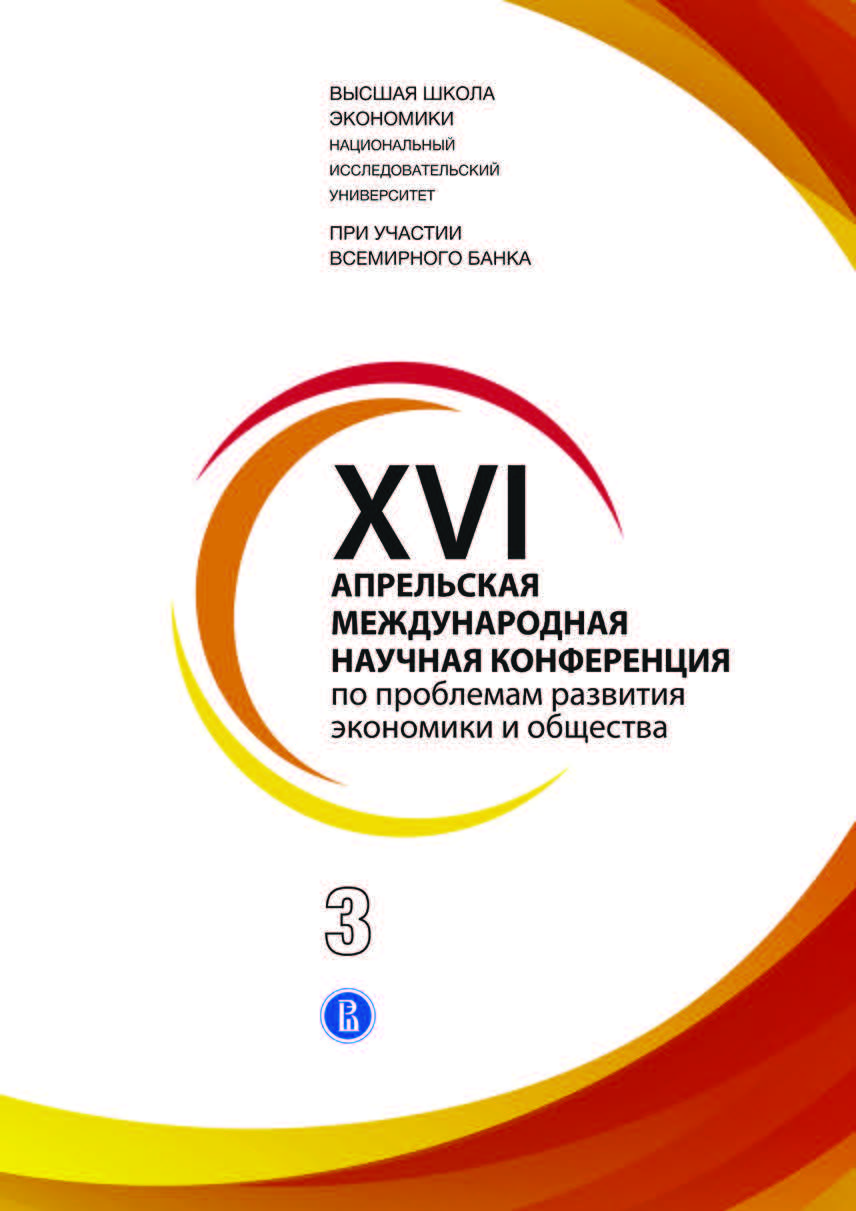• A
• A
• A
• ABC
• ABC
• ABC
• А
• А
• А
• А
• А
Regular version of the site

## On Evaluation of the Power Indices with Allowance of Agents’ Preferences in the Anonymous Games

P. 151-162.
Shvarts D., Ushakov M. N.

At calculation of the power indices, both well-known (Banzhaf, Shapley-Shubik and others and new (depending on the agent preferences) indices, one generally has to enumerate almost all coalitions, that is, the subsets of the set of players, which makes calculations impossible if the number of players exceeds fifty. Yet, if all players have an integer number of votes, there are players with the same number of votes, many coalitions have equal total number of votes or the sum of votes of all players is small, then the algorithms based on calculations using the generating functions become efficient. But these algorithms works only for classical power indices and some particular types of the power indices based on agents’ preferences. In this paper we consider an important specific case when all players have the same number of votes. For classical power indices in this case all players have the same power. However, it is not the case for the indices which allow preferences of agents. We introduce effective algorithms for calculation of the latter indices for most types of these indices.

### In bookEdited by: Е. Г. Ясин Кн. 3. М.: Издательский дом НИУ ВШЭ, 2016.